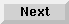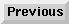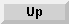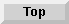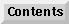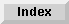10 Matrix Operations

## Arithmetic Operations

### Copying an Array

To copy the value of each element of an array into the corresponding element of the result array, use the MAT-copy statement. Syntax for this statement is as follows:

MAT result array = operand array

The two arrays must have the same number of dimensions. The number of elements in the result array must be greater than or equal to the number of elements in the operand array. For example:

```10   INTEGER C(4,4),D(3,3),E(4,2)
20   MAT D=(2.5)
30   MAT C=D
```
Each element of array D is set to 2.5, then the values of array D are copied into the elements of array C, then the working size of array C is redimensioned to be a 3 by 3 array.

```40   MAT E=C
```
If the above statement was included, an error would occur because the array E has only 2 elements in the second dimension while the operand array, C, has 3 elements.

Example for string array:

```50   DIM  A\$(5),B\$(5)
....

100  MAT  A\$=B\$
```

### Scalar Operations

The scalar operations allow an arithmetic or relational operation to be performed with each element of an array using a constant scalar. The result of the operation becomes the value of the corresponding element of the result array. Either of the following syntax statements may be used to perform scalar operations:

MAT result array = operand array operator (scalar)

MAT result array = (scalar) operand array operator

For example, the following program line multiplies each element in array C by 4 and stores the result in the corresponding elements of array B:

```30   MAT B=C*(4)
```
To further explain, take the following program line:

```150   MAT B=C>(100)
```
If any elements of array C have a value greater than 100, a value of 1 is entered in the corresponding elements of array B. A 0 is entered if the value of the element in array C is less than or equal to 100.

The following operators are allowed:

```+, -, *, /

=, < > or #, >, <, >=, <=
```
The two arrays must have the same number of dimensions. The number of elements in the result array must be greater than or equal to the number of dimensions in the operand array. The result array is redimensioned to the working size of the operand array after the operation.

### Matrix Arithmetic Operations

The MAT-arithmetic statement allows an arithmetic or relational operation to be performed with corresponding elements of two arrays. The result becomes the value of the corresponding element in the result array. Syntax for this statement is as follows:

MAT result array = operand array1 operator operand array2

For example, the following program line multiplies corresponding elements of arrays Hours and Rate and stores them in array Pay:

```200   MAT Pay=Hours.Rate
```
Note that a period (.) is used for multiplication, not an asterisk (\$\ast\$).

To further explain, take the following example:

```30   MAT A=B>C
```
If the value in the element of array B is greater than the corresponding element of array C, a value of 1 is stored in array A.

The following operators are allowed:

```+, -, /

=, < > or #, >, <, >=, <=
```
The result and operand arrays must have the same number of dimensions. The operand arrays must have the same number of elements in each dimension; the result array cannot have less.

Eloquence Language Manual - 19 DEC 2002